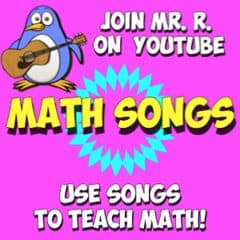# 2's Multiplication Facts Song!

LYRICS

Multiplying is real good
I learned my facts, I knew I could
Facts I’ve learned with strategies
Make multiplying real easy!

Makes numbers bigger my oh my,
Like greater than the sunny sky
Make multiplying real easy!

Everybody multiply,  multiply,  multiply
Everybody multiply by two!
Everybody multiply,  multiply, multiply
Everybody multiply by two

I learned them well, now I’m a pro,
There are no facts that I don’t know
I’ll use them now and way past college,
They’re really useful math time knowledge!

Multiplying is real good,
I learned my facts, I knew I could!
Facts I’ve learned with strategies
Make multiplying real easy!